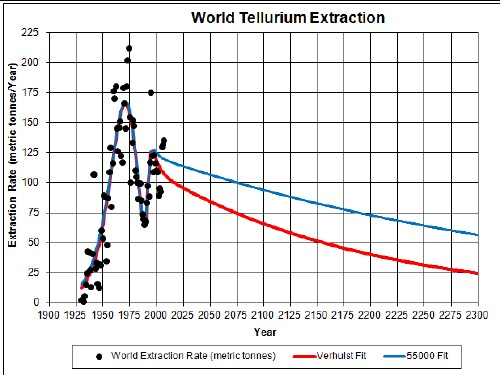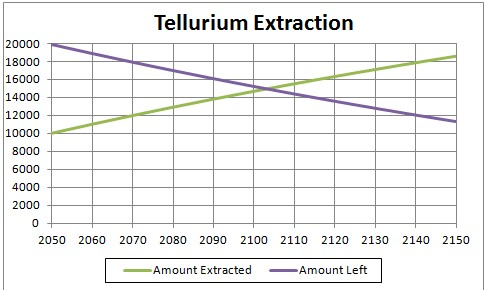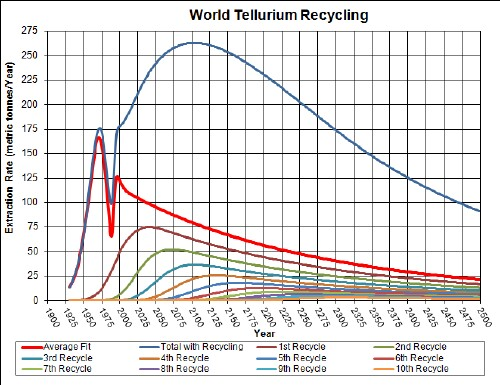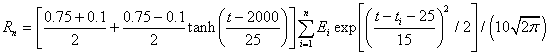# Tellurium Depletion Including Recycling

Tellurium is used in electronics, which makes it easy to recycle. The graph below shows the tellurium extraction data for the world and two Verhulst function fits to the data in order to extrapolate into the future.Tellurium extraction rate for the world and two Verhulst function fits to the data.

The red curve is a fit with total amount to be extracted of 29 x 103 tonnes, which incorporates the latest reserves estimate, and the blue curve is fit with total amount to be extracted of 55 x 103 tonnes, which incorporates the latest reserves-base estimate.

It appears that world-tellurium extraction is peaking at about or before now (2009).

Taking an average extraction curve of the two fits, the crossover point at year 2103 when the amount extracted is equal to the amount left to be extracted is shown here:## Recycling

Tellurium, especially the tellurium used to make ultracapacitors, could be recycled in the future. Assume that:

• The tellurium-extraction curve is the average of the two curves given above.
• Recycling of tellurium follows a hyperbolic tangent curve from 50% to 95% recycling with a break point of year 2000 and width 15 years.
• The recycling is delayed by a Gaussian curve peaking at a delay of 15 years and a width of 5 years.

The effective tellurium available for making items after the first ten recycling cycles is shown in the following graph, along with the effective cobalt available for each cycle:The equation for a recyling cycle is,

where Ei is the amount available from the previous cycle. Here is an example of the Excel coding:

{=((\$J\$2+\$I\$2)/2+((\$J\$2-\$I\$2)/2)*TANH((A27-\$K\$2)/\$L\$2))*SUM(\$I\$27:I27*(EXP(-1*((A27-\$A\$27:A27-\$N\$2)/\$O\$2)^2/2))/\$O\$2/SQRT(2*PI()))} (The curly bracket surrounding the term makes it into an array; it must be entered by holding down the SHIFT & CTRL keys while pressing the ENTER key.)

Of course, the recycling could be extended to more cycles, skewing the curve further into the future. However, the peak and fall off after it will not change because further cycles are essentally zero in that time region.

Thus, under the assumptions given above, the effective amount of tellurium available for making items peaks at about year 2100 and falls off rapidly after that. Humans will have taken concentrated tellurium deposits and scattered them across the surface of the earth.

The Excel spreadsheet is set up to make it easy to calculate with different recycling assumptions.

Minerals Depletion